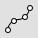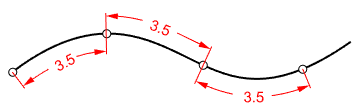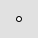# DivideCurve

Point Object >

Divide Curve by >

Number of Segments

Length of SegmentsHistory enabled

The Divide command creates point objects along a curve or splits a curve by equal length, equal chord length or number of segments.

#### Steps

1. Select curves.
2. Type the number of segments.

Or, click the Length or EqualChordLength option and enter a number.

Preview the result before pressing Enter.

Command-line options

##### Length

Divides a curve with the specified length.Length measurement starts from the natural start of a curve. Click the curve to start measuring from the other end.

##### EqualChordLengthDivides a curve with the specified straight distance (chord length).##### Split

Splits the curve instead of placing point objects.

##### MarkEnds

Places a point at the ends of the curve.

##### GroupOutput

Groups the resulting objects.

##### SubCrv

Type subcrv to select part of a curve as input.